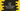# Java program to find the sum of all digits of a number### Java program to find the sum of all digits of a number :

In this tutorial, we will learn how to find the sum of all digits of a number in Java. Algorithm we are using is as below :

### Algorithm :

1. Start one infinite loop. This loop will run infinite times till user insert ‘-1’ as input.
2. Take the number from the user as input.
3. Declare one variable as ‘0’ to store the sum.
4. Using one ’while’ loop, get the modulo 10 of the number and add it to the sum. If the number is 123, get ‘123%10’ i.e. 3 and add it to sum.
5. Now, divide the number by 10 and set it as new value. i.e. for 123, set it to 123/10 = 12
6. Do this till the number become_ ‘0’._
7. Finally, Print out the result.

### Example program :

``````import java.util.Scanner;

public class Main {

private static void println(String message) {
System.out.println(message);
}

public static void main(String[] args) throws java.lang.Exception {
Scanner sc = new Scanner(System.in);

while (true) {
println("");
println("Enter a number ( -1 to exit ): ");

int no = sc.nextInt();

if (no == -1) {
break;
}
int sum = 0;

while (no > 0) {
sum += no % 10;
no = no / 10;
}

println("Sum of all numbers is " + sum);
}
}

}``````

### Example Output:

``````Enter a number ( -1 to exit ):
1
Sum of all numbers is 1

Enter a number ( -1 to exit ):
12
Sum of all numbers is 3

Enter a number ( -1 to exit ):
123
Sum of all numbers is 6

Enter a number ( -1 to exit ):
1234
Sum of all numbers is 10

Enter a number ( -1 to exit ):
12345
Sum of all numbers is 15

Enter a number ( -1 to exit ):
123456
Sum of all numbers is 21

Enter a number ( -1 to exit ):
-1

Process finished with exit code 0``````